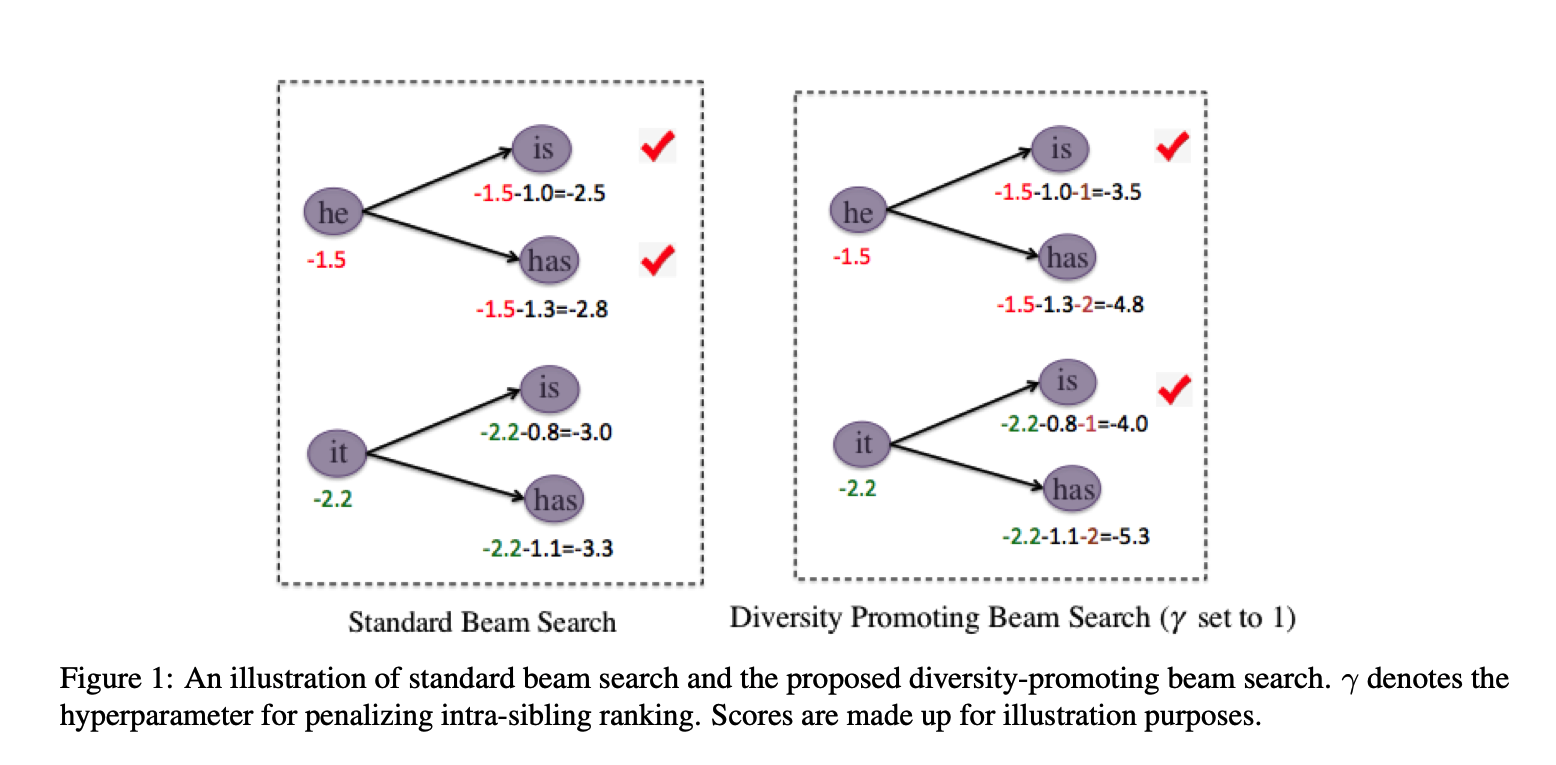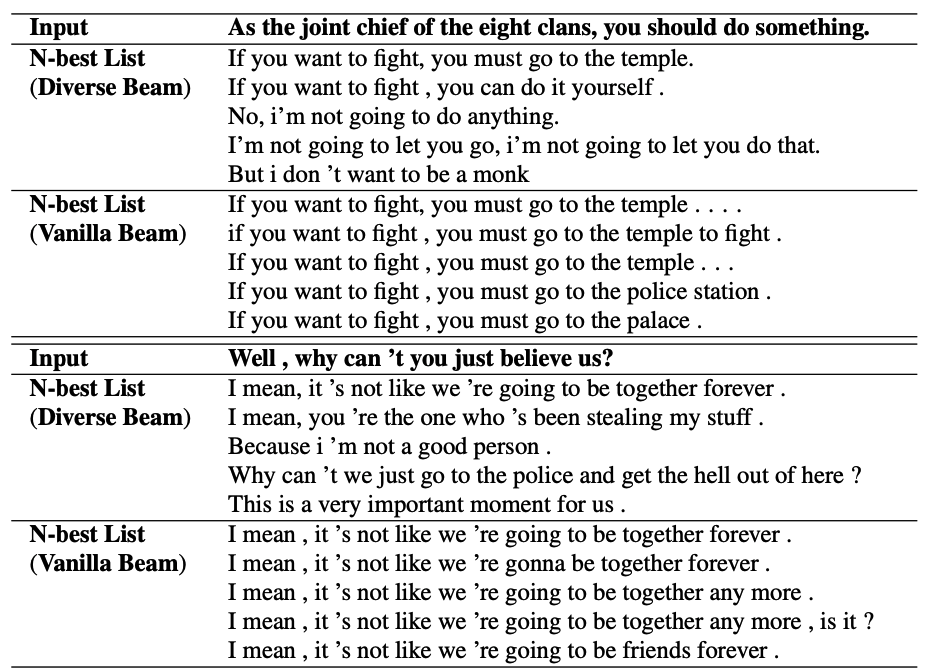Dice Loss for Data-imbalanced NLP Tasks
Improved Natural Language Generation via Loss Truncation
A Simple, Fast Diverse Decoding Algorithm for Neural Generation

## Dice Loss for Data-imbalanced NLP Tasks

https://arxiv.org/abs/1911.02855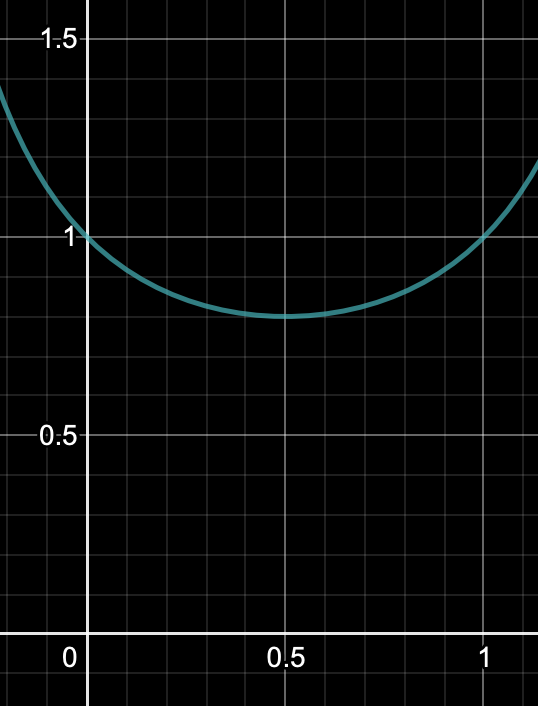class DiceLoss(nn.Module):
"""DiceLoss implemented from 'Dice Loss for Data-imbalanced NLP Tasks'
Useful in dealing with unbalanced data
"""

def __init__(self):
super(DiceLoss, self).__init__()

def forward(self, y_pred, y_true):
# shape(y_pred) = batch_size, label_num, **
# shape(y_true) = batch_size, **
y_pred = torch.softmax(y_pred, dim=1)
pred_prob = torch.gather(y_pred, dim=1, index=y_true.unsqueeze(1))
dsc_i = 1 - ((1 - pred_prob) * pred_prob) / ((1 - pred_prob) * pred_prob + 1)
dice_loss = dsc_i.mean()
return dice_loss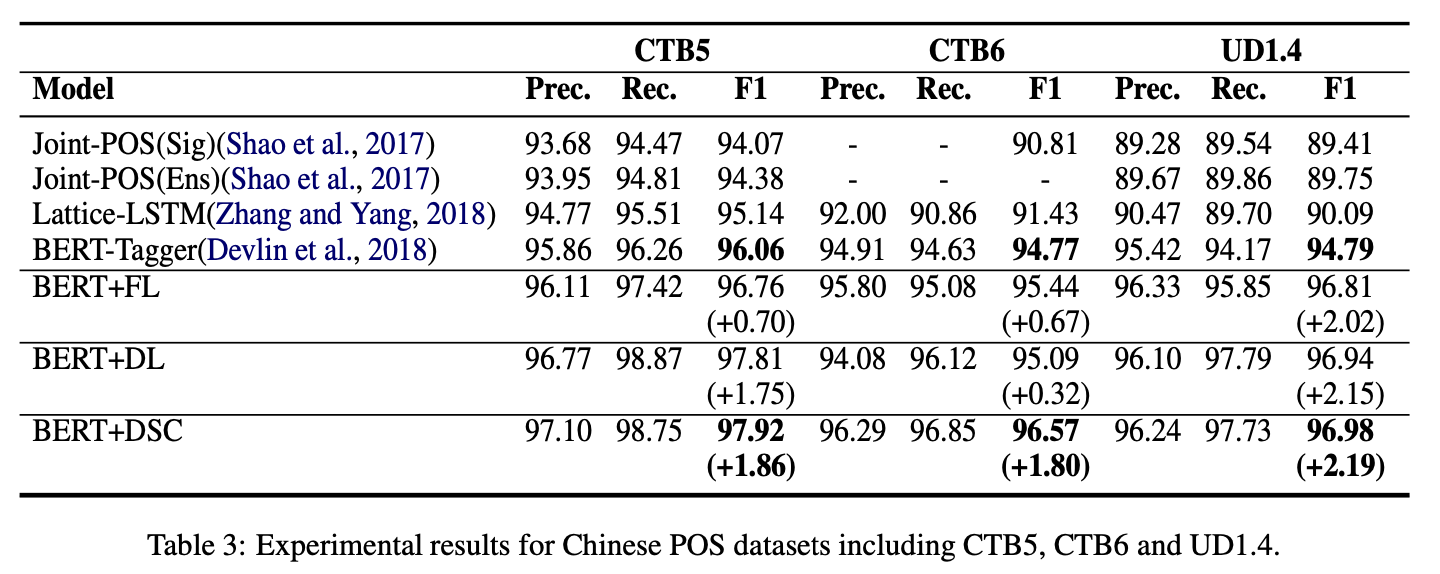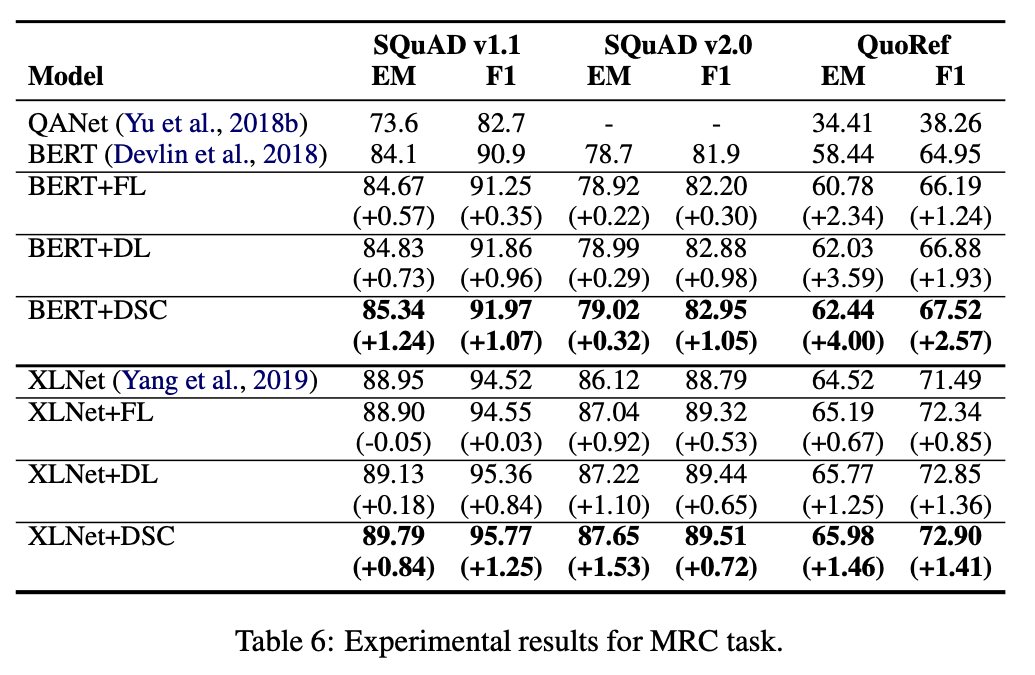• 比focal loss有更狠的懲罰，個人嘗試幾個資料集效果都不太好，還是需要根據資料的情況去使用啊。

## Improved Natural Language Generation via Loss Truncation

https://arxiv.org/abs/2004.14589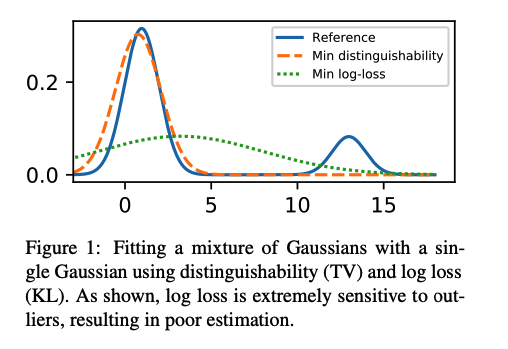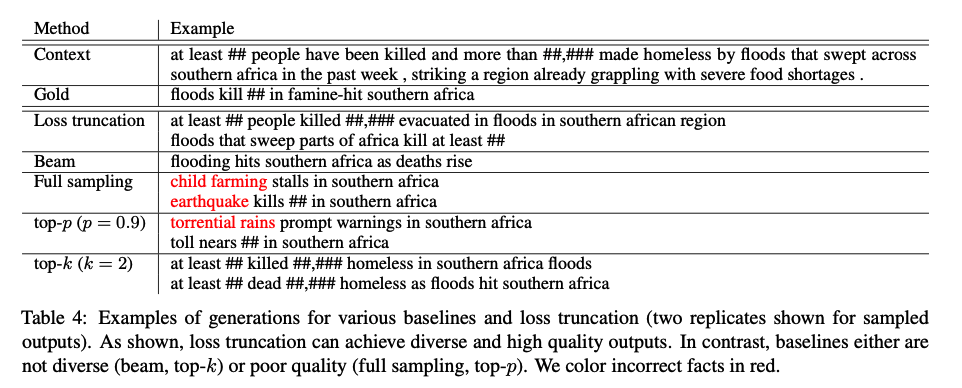## A Simple, Fast Diverse Decoding Algorithm for Neural Generation

https://arxiv.org/pdf/1611.08562.pdf

Beamsearch的結果不夠多元化，很多時候是前文句子一樣，後文稍有變化，導致整體看起來變化不大。